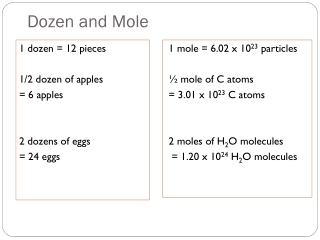DownloadDownload PresentationDozen and Mole

# Dozen and Mole

Télécharger la présentation## Dozen and Mole

- - - - - - - - - - - - - - - - - - - - - - - - - - - E N D - - - - - - - - - - - - - - - - - - - - - - - - - - -
##### Presentation Transcript

1. Dozen and Mole 1 mole = 6.02 x 1023 particles ½ mole of C atoms = 3.01 x 1023 C atoms 2 moles of H2O molecules = 1.20 x 1024 H2O molecules 1 dozen = 12 pieces 1/2 dozen of apples = 6 apples 2 dozens of eggs = 24 eggs

2. Mole • amount of substance containing the same number of particles as 12 g of C-12. • 1 mole contains 6.02 x 1023 particles (Avogadro’s number) Particles – may be atoms, molecules, ions

3. Mole and Number of Particles • 1 mole of any substance contains 6.02 x 1023 particles (atoms, molecules, ions) 1 mole C-12 6.02 x 1023 atoms 1 mole CO2 6.02 x 1023 molecules

4. Mole – Number of Particles Conversion x 6.02 x 1023 particles/mole Mole Number of Particles Sample Problem 1: How many molecules are there in 0.25 moles of H2O? 0.25 mol H2O x 6.02 x 1023 molecules H2O mol = 1.505 x 1023 molecules H2O

5. Mole – Number of Particles Conversion Mole Number of Particles / 6.02 x 1023 particles/mole Sample Problem 2: How many moles are there in 1.2 x 1024 molecules of H2O? 1.2 x 1024 molecules H2O x 1 mole H2O 6.02 x 1023 molecules H2O = 2.0 moles H2O

6. Molar Mass 1 mole C-12 6.02 x 1023 atoms 12 g 1 mole CO2 6.02 x 1023 molecules 44 g

7. Molar Mass What is the molar mass of Mg(OH)2? Mg H O 1 mole 2 moles 2 moles 1(24g) = 24 g 2 (1g) = 2g 2 (16g) = 32g 24g+ 2 g +32 g = 58 g The molar mass of Mg(OH)2 is 58g.

8. Mole –Mass Conversion / Molar Mass Mass Mole x Molar Mass Sample Problem 1: What is the mass of 0.25 moles of H2O? Mass of H2O = 0.25 mol x 18 g mol = 4.5 g

9. Mole –Mass Conversion / Molar Mass Mass Mole x Molar Mass Sample Problem 2.How many moles are there in 36 g of H2O? Moles of H2O = 36 g x 1 mol 18g = 2.0 mol